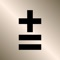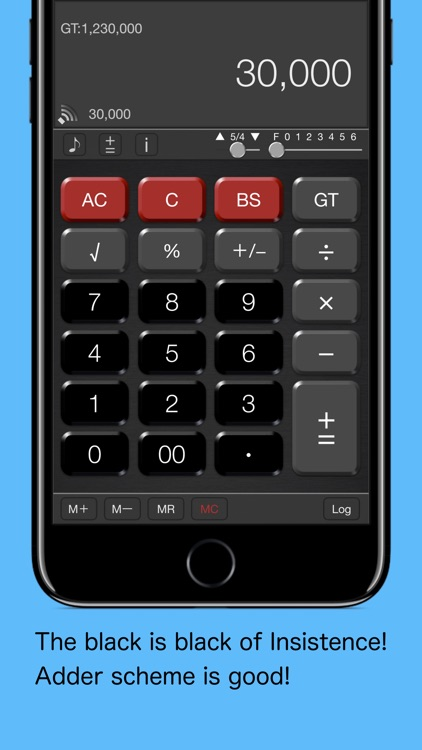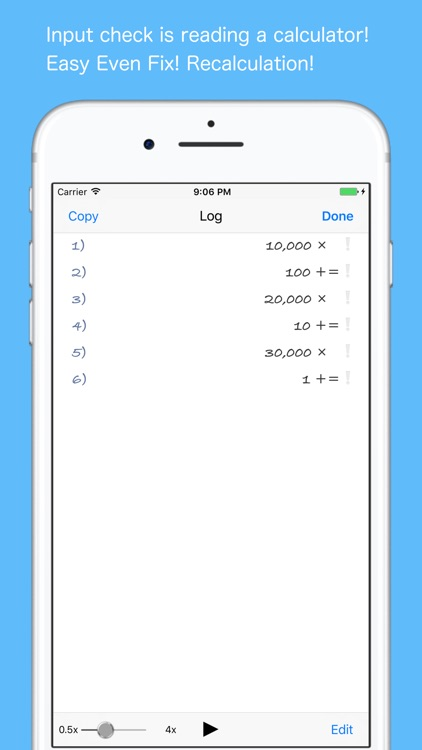## Do you know the calculator of optimal adder method for an accounting calculationby Shinichi AokawaDo you know the calculator of optimal adder method for an accounting calculation?### App Details

Version
14.2
Rating
NA
Size
15Mb
Genre
Productivity Utilities
Last updated
October 25, 2020
Release date
October 5, 2012

### App Screenshots### App Store Description

Do you know the calculator of optimal adder method for an accounting calculation?
(with the calculation method change function)
Try it on the iPhone and iPad you always carry around.

【Feature】
- This can switch an "adder calculator" and "ordinary calculator”
- Simple setting of the design of the calculator
- Sound effects
- Input log and copy
- Input log editing + recalculation function
- Voice reading of the input log (for iOS7)
- 14 Number of significant digits
- Always display "Grand Total"
- Always display "Memory"
- BackSpace function

Simple design that is emphasis in the adder calculation function

【How to use an adder calculator】
”(10×10)+(5×5)-(6×5)=95”
10 [×] 10 [+=] 5 [×] 5 [+=] 6 [×] 5 [-] [GT]

Ver5.5.3 later
% Calculation example : 500 + (500 × 12%) = 560
500 [+=] 12 [%] [+=]
(Old : 500 [+=] [×] 12 [%] [+=])

【How to use an ordinary calculator】
”(10×10)+(5×5)-(6×5)=95”
10 [×] 10 [=][M+] 5 [×] 5 [=][M+] 6 [×] 5 [=] [M-][MR]
Added to the Grand Total by "="

The continuous input will be "10 × 10 + 5 × 5 - 6 × 5 = 2595"

Ver5.5.3 later
% Calculation example : 500 + (500 × 12%) = 560
500 [+] 12 [%] [=]
(Old : 500 [+] [×] 12 [%] [=])

Disclaimer:
AppAdvice does not own this application and only provides images and links contained in the iTunes Search API, to help our users find the best apps to download. If you are the developer of this app and would like your information removed, please send a request to [email protected] and your information will be removed.